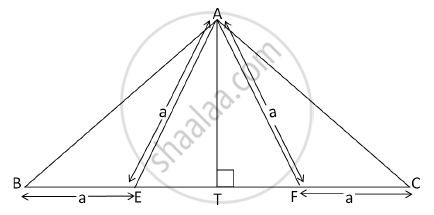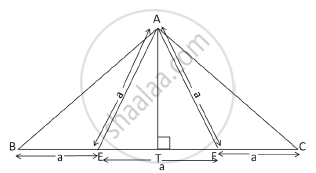# In the following figure, AE = EF = AF = BE = CF = a, AT ⊥ BC. Show that AB = AC = √3×a - Geometry

Sum

In the following figure, AE = EF = AF = BE = CF = a, AT ⊥ BC. Show that AB = AC =  sqrt3xxa#### Solution

Given: AE = EF = AF = BE = CF,
AT ⊥ EF
ΔAEF is equilateral triangle.

ET = TF =a/2

BT = CT = a +a/2....(1)In right triangles, ΔATB and ΔATC,

AT = AT … (Side common to both triangles)
∠ATB = ∠ATC … (Right angles)
BT = CT …. (from 1)
∴ ΔATB ≅ ΔATC …..(by SAS)
∴ AB = AC
In ΔAEF, AE = AF = EF …(Given)
∴ ΔAEF is an equilateral triangle.

AT =sqrt(3)/2 a ...(Altitude of equilateral triangle)

In ΔATB, (AB)^2=(AT)^2+(BT)^2

(AB)^2=(sqrt(3)/2a)2+(a+a/2)^2=(3a^2)/4+(9a^2)/4=(12a^2)/4=3a^2

AB=sqrt(3)a

i.e.,AB = AC =sqrt(3)a

Concept: Similarity in Right Angled Triangles
Is there an error in this question or solution?